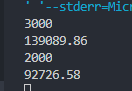2021-05-13 20:58

# 麻烦大佬们帮我看看这道题的问题

#include<stdio.h>
int main()
{
int m;
while(scanf("%d",&m)!=EOF)
{
double sum=m;
for(int i=47;i>0;i--)
{
sum= (sum+m)/(1 + 0.0171/12);
}
printf("%.2lf\n",sum);
}
return 0;
}

3000

2000

139089.86

92726.58

• 写回答
• 好问题 提建议
• 追加酬金
• 关注问题
• 邀请回答

#### 3条回答默认 最新

•最佳回答

你最后一个月sum=m是直接赋值给sum了，没有算利息。

你输入3000数据是139093.86也不对，

样例输出是 139089.86

正确应该是:

``````#include<stdio.h>
int main()
{
int m;
while(scanf("%d",&m)!=EOF)
{
double sum=0;
for(int i=48;i>0;i--)
{
sum= (sum+m)/(1 + 0.0171/12);
}
printf("%.2lf\n",sum);
}
return 0;
}``````评论
解决 无用
打赏 举报